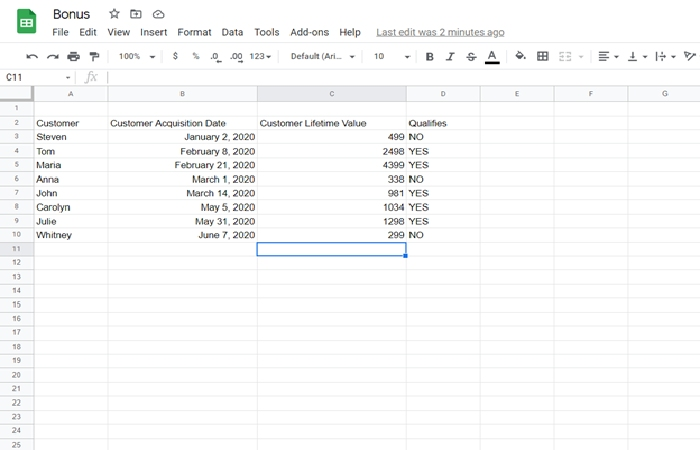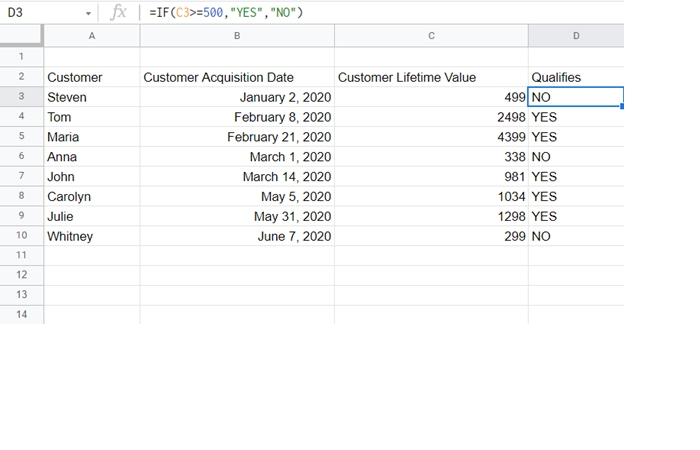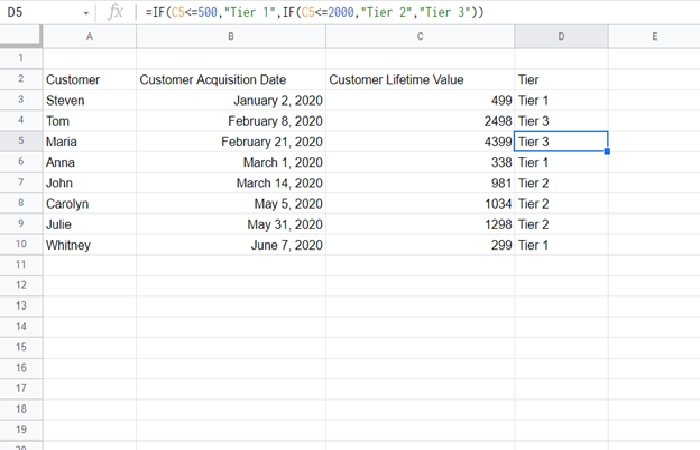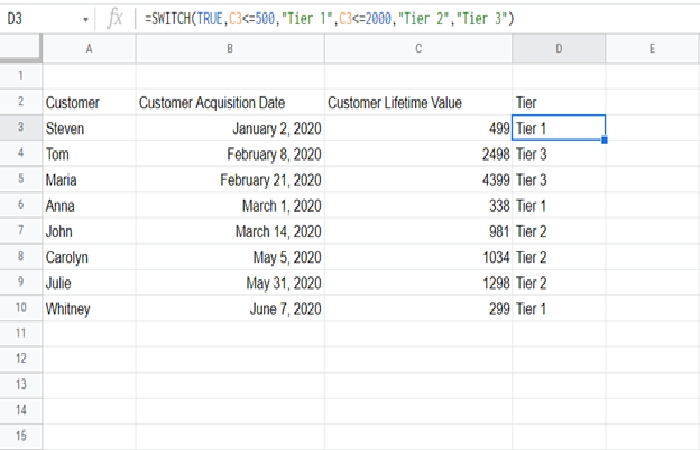Home » Blog » Finance » The Power of the IF Function in Finance

# The Power of the IF Function in Finance

0 comment

IF Function – Every programming language has an “IF” statement. An IF statement is a type of logical statement that makes it possible to control the flow of your algorithm. And IF that doesn’t make sense, we’ll explain it in clearer terms below.

The “IF” statement may take some time to learn. But once you understand how the IF function works, you’ll be able to create very complicated spreadsheets.

Let’s take a deeper look at the IF function.

## How Does the IF Function Work?

Consider an example. We have a list of customers, their acquisition dates, and their lifetime value. Currently, we’re running a new promotion. Any customer who has spent over \$500 will get a bonus to spend.The desired result is above. Wherever the Customer Lifetime Value is over \$500, we want the bonus section to say “YES.”

### We do this with the “IF” statement:Let’s break this down.

C3>=500

This means that the “Customer Lifetime Value” column is MORE THAN or EQUAL TO 500. Other operators that you might use would be:

• <=, less than or equal to.
• >, more than.
• <, less than.
• ==, equal to.
• !=, not equal to.

These comparatives are consistent throughout many programming languages.

Next is:

“YES”

If the prior statement was true, then it will print “YES.”

Next is:

“NO”

If the prior statement was false, then it will print “FALSE.”

### Deeper Into the IF Statement

So, the IF statement creates a logical branch. It asks you whether a certain statement is true or false (such as “CTV <= 500”). If it’s true, it does the first thing. If it’s false, it does the second thing.

Let’s say instead of a bonus, we wanted to separate our customers into tiers.

\$0 to \$500 of spending: Tier 1

\$501 to \$2,000 of spending: Tier 2

\$2,001+ of spending: Tier 3This merits some explanation. Here, we actually nested the IF commands.

=IF(C5<=500,”Tier 1”,IF(C5<=2000,”Tier 2”,”Tier 3”))

In plain language, this says:

If the customer has spent less than or equal to \$500, print Tier 1.

Otherwise, if the customer has spent less than or equal to \$2,000, print Tier 2.

Otherwise, print Tier 3.

Note that the above essentially prints “Tier 3” for anyone who is less than or equal to \$2,000 in purchasing.

The value of the IF function in finance is that it can easily tell you whether certain values are between specific parameters.

And that’s not all the IF function can do. The IF function could also be used to do things such as detect whether a given value is a number or a string, or detect whether a column has been left null.

## The SWITCH Function

Above, we used the IF function as a nested function. But another common alternative is using the SWITCH function. The SWITCH function is essentially a list of parameters and responses. In the above example, we would have instead done:This option is a little more readable. In SWITCH, you enter in pairs.

C3<=500         Tier 1

C3<=2000       Tier 2

Otherwise       Tier 3

SWITCH can be a little easier to read if you find it difficult to read nested IF statements (IF statements inside of themselves).

IF and SWITCH are both logical functions. They use machine logic to determine what to print. They are the building blocks of all programming and they can be very useful for anyone to learn to use.